Get our FREE VBA eBook of the 30 most useful Excel VBA macros.

Automate Excel so that you can save time and stop doing the jobs a trained monkey could do.

# Top 10 with formulas in Excel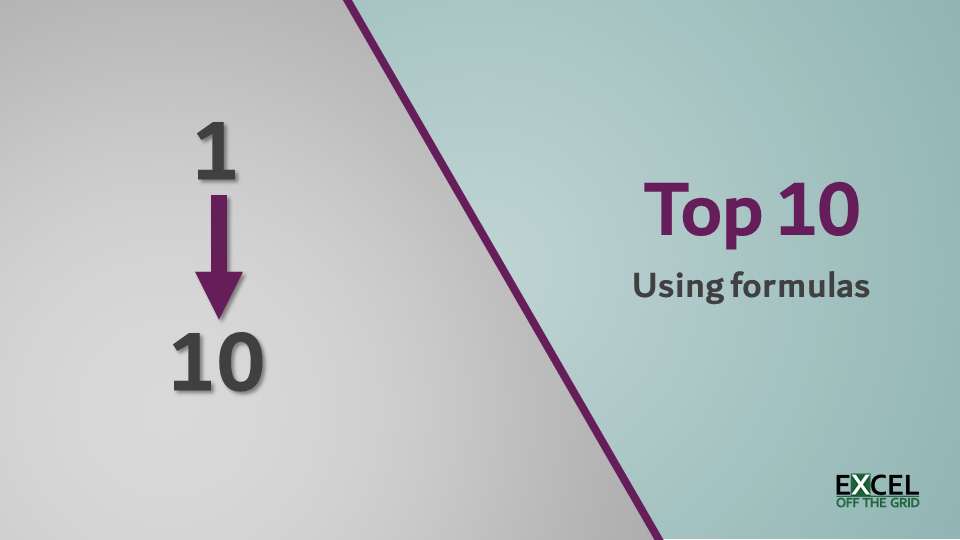A top 10 list is a common form for displaying information, especially on dashboards and summarized reports.  It is easy to create the list when working with sorted data, just cell link to the top 10 items in the list… easy!  It’s also relatively simple when using Auto Filter, Tables and Pivot Tables, as it is a default filter setting within these features.  However, when creating a top 10 with formulas on a non-sorted dataset, things become a little bit tricky.

In this post, I will show you exactly how to do it.  Through these methods, you’re not restricted to a top 10; you can create a top 5, top 8, or any number you choose.

Some of the most common formula problems with top 10 lists are:

• Dealing with duplicate values
• Working with categories
• How to change it to get the bottom 10

Don’t worry, we will cover all of these (they are also all included in the example file).  Hopefully, along the way, you’ll learn a little more about how Excel works.

#### Dynamic arrays

Users with an Office 365 subscription now have access to a group of functions, which make use of the new dynamic array calculation engine.  These give us a nice simple way to calculate a top 10.  So, if you have a dynamic array enabled version of Excel, then be sure to check out that section.

I recommend you download the example file for this post.  Then you’ll be able to work along with examples and see the solution in action, plus the file will be useful for future reference.

Note: If you don’t have a Dynamic Array enabled version of Excel, the tabs containing those examples will display errors.

Watch the video:

Watch the video on YouTube

Contents

We are going to start with traditional functions (i.e., non-dynamic array).  Using these, it is easy to obtain the top 10 values.  But getting the names/labels which relate to those ten values is the challenge.

Start by looking at the Top 10 – non-DA tab of the example file.

### Using the LARGE function

In our example file, there are 26 customers, with their values.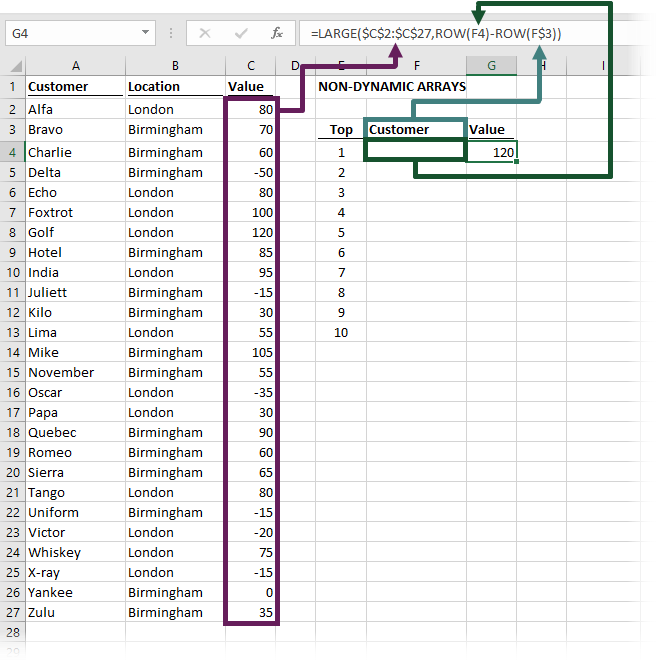If we wanted to create a top 10 of these customers (without sorting the list), we could use the LARGE function.  Cell G4 contains this formula:

`=LARGE(\$C\$2:\$C\$27,ROW(F4)-ROW(F\$3))`

LARGE only has two arguments:

`=LARGE(Range, k)`
• Range – The range of data to be analyzed.
• k – The nth item to be found.

In our example, the data range to analyze is cells C2 to C27.

The k value is calculated as the row number, minus the row number of the header row.  This always calculates the relative row position in a range of cells.  The first row of data calculates as 1, the second row, calculates as 2, and so on.

`ROW(F4)-ROW(F\$3)`

The formula in cell G4 has been copied down to display the top 10 values.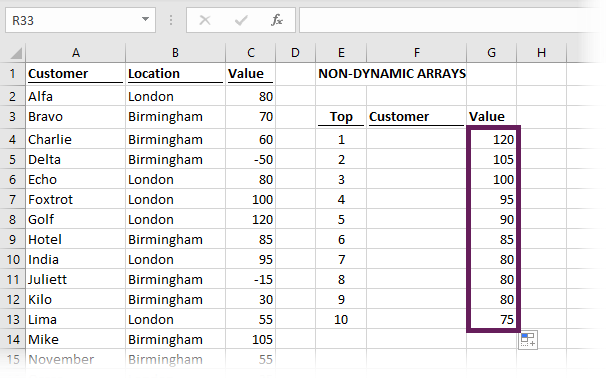### Finding the labels for the top 10

We have the values, so now we can calculate the customer name for that value.

We can’t use VLOOKUP as the customer name is to the left of the lookup value.  Instead, we will use the INDEX/MATCH formula combination.  Cell F4, in our example, would contain the following formula.

`=INDEX(\$A\$2:\$A\$27,MATCH(G4,\$C\$2:\$C\$27,0))`

If this formula were copied down into cells F5 – F13.  Our worksheet would display as follows:There is one huge problem – our top 10 values are not unique; there are 3 values of 80 (see the screenshot above).  A basic INDEX/MATCH only returns the first value, so it finds the name Alfa 3 times.  Yet, Echo and Tango, who also have 80, do not feature on the list.  This is clearly an error, so how do we get around this?

### Finding the labels when the top 10 values are not unique

To solve the issue of finding labels with non-unique values, we will turn to an advanced array formula.  The formula in cell F4 should be:

```{=INDEX(\$A\$2:\$A\$27,SMALL(IF(\$C\$2:\$C\$27=G4,ROW(\$C\$2:\$C\$27)-ROW(\$C\$1)),
COUNTIF(\$G\$4:G4,G4)))}```

Woah!!! That’s big and complicated!.

This is a special type of formula, known as an array formula.  Don’t put the curly brackets ( {  } ) at the start or the end when typing the formula into the formula bar; when you press Ctrl+Shift+Enter, Excel will add these itself.  Pressing Ctrl+Shift+Enter lets Excel know that it is an array formula.REMEMBER! – If you go back into an array formula to edit it, you need to press Ctrl + Shift + Enter again to re-enter the formula.

This formula is like INDEX/MATCH, but returns the 1st, 2nd, 3rd, 4th… or nth value.  Let’s dig a bit deeper to understand how it works.

#### Section 1 – IF function

In the middle of the formula, we find an IF function.

`IF(\$C\$2:\$C\$27=G4,ROW(\$C\$2:\$C\$27)-ROW(\$C\$1))`

In English, this says:

If C2 = G4 then return the count of rows between C2 and C1.

As this is an array formula, it automatically goes onto the next row and calculates again

If C3 = G4 then return the count of rows between C3 and C1.

And it keeps on going

If C4 = G4 then return the count of rows between C4 and C1.

This will go all the way from C2 to C27.

For the formula in F4, the IF function calculates as:

```{FALSE;FALSE;FALSE;FALSE;FALSE;FALSE;7;FALSE;FALSE;FALSE;FALSE;FALSE;FALSE;
FALSE;FALSE;FALSE;FALSE;FALSE;FALSE;FALSE;FALSE;FALSE;FALSE;FALSE;FALSE;FALSE}```

The 7th item in the list matches cell G4; therefore, the only value which is not FALSE is 7.

#### Section 2 – SMALL and COUNTIF functions

If we feed this into the SMALL function, it would be as follows:

```SMALL({FALSE;FALSE;FALSE;FALSE;FALSE;FALSE;7;FALSE;FALSE;FALSE;FALSE;FALSE;FALSE;
FALSE;FALSE;FALSE;FALSE;FALSE;FALSE;FALSE;FALSE;FALSE;FALSE;FALSE;FALSE;FALSE},
COUNTIF(\$G\$4:G4,G4))```

SMALL finds the nth smallest value.  It works in a similar way to LARGE, but for the smallest value.

SMALL only has two arguments:

`=SMALL(Range,k)`
• Range – The range of data to be analyzed.
• k – The nth item to be found.

In this context, COUNTIF calculates how many instances of the value have appeared in the top 10 already.

For the first row, there is only 1 item in the top 10 with a score of 120, so COUNTIF will calculate as 1.

The SMALL will find the first smallest value, which is 7, as all the other results are FALSE.

Finally, let’s wrap that in the INDEX function to find the 7th value in the source table.

`=INDEX(\$A\$2:\$A\$27,7)`

This calculates to cell A8, which in our example is Golf.

All the workings above were to show how the formula works.  Now we can copy the complete formula down into cells F5 – F13.

#### Testing on duplicate values

The first row does not have a duplicate value.  So, let’s test it out with cell F11.  There are 3 values in the top 10 which are all 80; they are found in G10, G11 and G12.  The label in F11 should be Echo, as it is the 2nd value of 80.

The formula in F11 is:

```{=INDEX(\$A\$2:\$A\$27,SMALL(IF(\$C\$2:\$C\$27=G11,ROW(\$C\$2:\$C\$27)-ROW(\$C\$1)),
COUNTIF(\$G\$4:G11,G11)))}```

The IF portion of the function calculates as:

```{1;FALSE;FALSE;FALSE;5;FALSE;FALSE;FALSE;FALSE;FALSE;FALSE;FALSE;FALSE;
FALSE;FALSE;FALSE;FALSE;FALSE;FALSE;20;FALSE;FALSE;FALSE;FALSE;FALSE;FALSE}```

Rows 1, 5, and 20 in the source table all match the value of 80.

The COUNTIF calculates to 2, which makes sense, as it is the 2nd matching value we are looking for.

```SMALL({1;FALSE;FALSE;FALSE;5;FALSE;FALSE;FALSE;FALSE;FALSE;FALSE;FALSE;FALSE;
FALSE;FALSE;FALSE;FALSE;FALSE;FALSE;20;FALSE;FALSE;FALSE;FALSE;FALSE;FALSE},2)```

As the second smallest number is 5, the INDEX function returns the 5th value in the source list, which is Echo.

Fantastic stuff, right!!!

### Bottom 10 values

If you want the bottom 10 values, the only change is that the formula to get the values uses SMALL, rather than LARGE.

Look at the example file.  The formula in cell G18 is:

`=SMALL(\$C\$2:\$C\$27,ROW(F18)-ROW(\$F\$17))`

The only difference between cell G18 and cell G4 is the use of the SMALL function.

The formula in cell F18 is the same as we saw above before (but pointing at different cells).

```=INDEX(\$A\$2:\$A\$27,SMALL(IF(\$C\$2:\$C\$27=G18,ROW(\$C\$2:\$C\$27)-ROW(\$C\$1)),
COUNTIF(\$G\$18:G18,G18)))```

Sometimes we want to calculate the top 10 results where a specific condition is met.  In our example file, look at the Top 10 – non-DA with criteria tab.

Cell J4 contains the name of the city; London or Birmingham.  The top 10 will only be returned for customers in that city.Cell G4 has the following function:

`{=LARGE(IF(\$B\$2:\$B\$27=\$J\$4,\$C\$2:\$C\$27),ROW(F4)-ROW(F\$3))}`

This is another array formula.  Remember, don’t enter the curly brackets, but do press Ctrl+Shift+Enter again.

This uses the same logic as we’ve already seen.  The IF function checks cells B2 to B27; if the value matches cell J4, the value in C2 to C27 is returned.

When the city is London, the IF calculate as follows:

```{80;FALSE;FALSE;FALSE;80;100;120;FALSE;95;FALSE;FALSE;55;FALSE;FALSE;
-35;30;FALSE;FALSE;FALSE;80;FALSE;-20;75;-15;FALSE;FALSE}```

By using the LARGE function, only the London values will be returned into the top 10.  All the non-London values calculate as FALSE.

We make a similar adjustment to the formula which calculates the customer name (the added section is in bold)

```={INDEX(\$A\$2:\$A\$27,SMALL(
IF((\$C\$2:\$C\$27=G4)*(\$B\$2:\$B\$27=\$J\$4),ROW(\$C\$2:\$C\$27)-ROW(\$C\$1)),
COUNTIF(G4:\$G\$4,G4)))}```

This is an array formula.  Don’t enter the curly brackets.  Do press Ctrl+Shift+Enter again.

This uses similar TRUE/FALSE logic to only calculate the values which match the city selected.

Look at the example file (Cells E17 – H27) if you want to see how a bottom 10 with criteria is calculated.## Dynamic array functions

Everything above seems quite complicated.  Wouldn’t it be better if we could use some more straightforward formulas?  If you have a dynamic array enabled version of Excel (only available to Office 365 subscribers at the time of writing), then you’re in luck.

### INDEX / SORT / SEQUENCE function combination

Look at the Top 10 DA tab of the example file.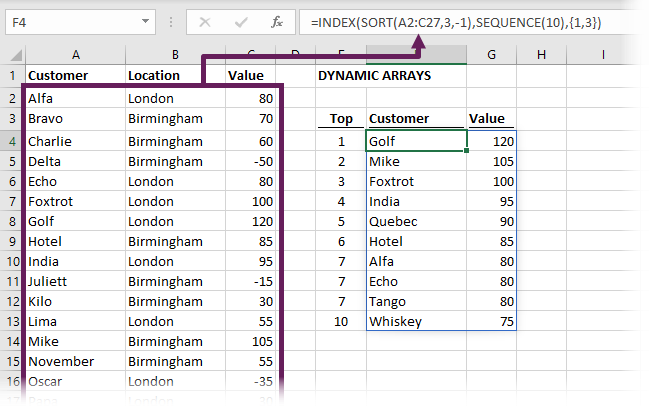In cell F4 the formula is:

`=INDEX(SORT(A2:C27,3,-1),SEQUENCE(10),{1,3})`

And that’s it!  No more array formulas required for the top 10, no need to press Ctrl+Shirt+Enter, no need to copy down.

Let’s dig into this a bit deeper.

#### SORT

SORT has four arguments:

`=SORT(array, [sort_index], [sort_order], [by_col])`
• array: The range of cells, or array of values to be sorted.
• [sort_index]: The nth column or row to apply the sort to.  For example, to sort by the 3rd column, the sort index would be 3.
• [sort_order]: 1 = sort in ascending order, -1 = sort in descending order (if excluded the argument will default to 1).
• [by_col]: TRUE = sort by columns, FALSE = sort by rows (if excluded the argument will default to FALSE).

In our formula, we used the following SORT.

`SORT(A2:C27,3,-1)`

This sorts the cells A2 to C27 on the 3rd column in descending order.

Find out more about the SORT function in this article: SORT Function in Excel

#### SEQUENCE

The SEQUENCE function has four arguments:

`=SEQUENCE(Rows, [Columns], [Start], [Step])`
• Rows: The number of rows to return
• [Columns]: The number of columns to return.  If excluded, it will return a single column.
• [Start]: The first number in the sequence.  If omitted, it will start at 1.
• [Step]: The amount to increment each subsequent value.  If excluded, each increment will be 1.

In our example, the following creates a list from 1 to 10.  We only need the first argument, as we can use the default options for the optional arguments.

`SEQUENCE(10)`

Find out more about the SEQUENCE function in this article: SEQUENCE function in Excel

#### INDEX

Let’s put both SORT and SEQUENCE into a traditional INDEX function:

`=INDEX(SORT(A2:C27,3,-1),SEQUENCE(10),{1,3})`

The formula returns the first 10 results from the SORT and returns columns 1 and 3.

That was so easy… right.

### Bottom 10

To get the bottom 10, we only need to change the 3rd argument of the SORT function from -1 to 1.  Look at cell F18 in the example file to see this in action.

### Dynamic arrays with criteria

Even if we have criteria to apply, it is still straight forward with dynamic arrays.

Now, look at the Top 10 – DA with criteria tab in the example file.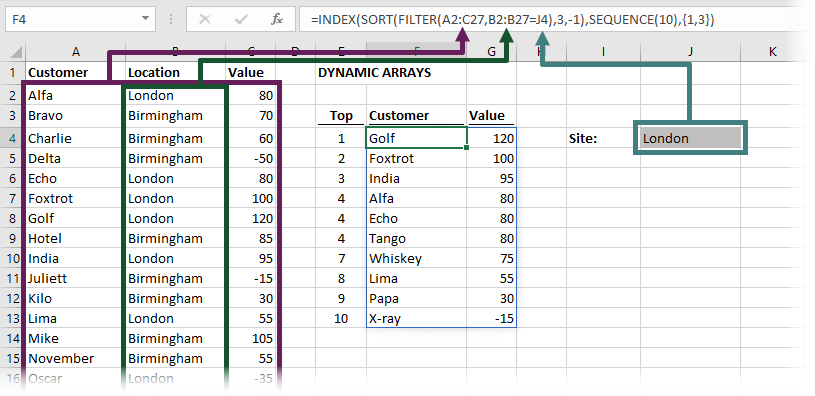The formula in Cell F4 is:

`=INDEX(SORT(FILTER(A2:C27,B2:B27=J4),3,-1),SEQUENCE(10),{1,3})`

The only difference to the previous example is that we are using the FILTER function to only include the matching items, before it is fed into the SORT function.

#### FILTER

FILTER has three arguments:

`=FILTER(array, include, [if_empty])`
• array: The range of cells, or array of values to filter.
• include: An array of TRUE/FALSE results, where the TRUE values will be retained in the filter.
• [if_empty]: The value to display if no rows are returned.

In our example, the FILTER function is:

`FILTER(A2:C27,B2:B27=J4)`

It is returning cells A2 to C27, but only where the values from B2 to B27 equals the selected city in cell J4.

Find out more about the FILTER function in this article: FILTER function in Excel

## Conclusion

Once you know the techniques and the formulas, calculating a top 10 using formulas in Excel isn’t too bad.

This post demonstrates how good dynamic arrays are; we don’t need to rely on complex array formulas any longer.Get our FREE VBA eBook of the 30 most useful Excel VBA macros.

Automate Excel so that you can save time and stop doing the jobs a trained monkey could do.

Don’t forget:

If you’ve found this post useful, or if you have a better approach, then please leave a comment below.

Do you need help adapting this to your needs?

I’m guessing the examples in this post didn’t exactly meet your situation.  We all use Excel differently, so it’s impossible to write a post that will meet everybody’s needs.  By taking the time to understand the techniques and principles in this post (and elsewhere on this site) you should be able to adapt it to your needs.

But, if you’re still struggling you should:

1. Read other blogs, or watch YouTube videos on the same topic.  You will benefit much more by discovering your own solutions.
2. Ask the ‘Excel Ninja’ in your office.  It’s amazing what things other people know.
3. Ask a question in a forum like Mr Excel, or the Microsoft Answers Community.  Remember, the people on these forums are generally giving their time for free.  So take care to craft your question, make sure it’s clear and concise.  List all the things you’ve tried, and provide screenshots, code segments and example workbooks.
4. Use Excel Rescue, who are my consultancy partner.   They help by providing solutions to smaller Excel problems.

What next?
Don’t go yet, there is plenty more to learn on Excel Off The Grid.  Check out the latest posts:

## 44 thoughts on “Top 10 with formulas in Excel”

1.Mike says:

That’s really impressive. Thanks for this and the step-by-step explanation. It works great for me.

•Excel Off The Grid says:

Thanks Mike – I’m glad it was useful.

2.Hosseini says:

Thanks

3.Rene Garcia says:

Very good post, thank you!

•Excel Off The Grid says:

🙂 Thanks Rene.

4.Patrick Whelan says:

Holy moley! My head is smoking. My goal is to:
Do a CoViD-19 CFR by country, graphic the top 10, once daily, with auto update from the Johns Hopkins map on the web. Then create a follow on line graph that also updates daily after the numbers come in.
Lol, Not even sure all of that is possible, but not seeing the info online and it’s important to compare progress to other countries, so I was using the build idea to explore and try to learn.
Obviously bit off way more than I can chew by myself.
But thank you for such details, I’m reading it all 5 times over.
Smiles, Patrick

•Excel Off The Grid says:

That sounds like an interesting project. Hopefully this post will meet your needs.

5.James says:

Very nice!

Thanks again Mark!!

6.Juan Carlos Castillo says:

Great post, Im going to verify this page for future research

7.Deven says:

This is the best and most comprehensive post I’ve come across. One post that answered all my questions. All had to do was scroll down to see if there were answers to my follow up questions.

5 Stars!!!

•Excel Off The Grid says:

Thank you Deven – very kind of you to say.

8.Jeremy David Weckwerth says:

should this ROW(F4)-ROW(F\$3) be E4 and E\$3?

•Excel Off The Grid says:

It actually doesn’t matter which column it is. The result is the same, as ROW(F4) and ROW(E4) both calculate to 4.

9.Denise says:

How can I do the thing but with multiple criteria?

•Excel Off The Grid says:

I’m assuming you’re referring to the traditional Excel functions. In which case, using the example file. If you have an additional category in cells D2:D27 and the condition in Cell J5, the formula in G4 would be:

`{=LARGE(IF((\$B\$2:\$B\$27=\$J\$4)*(\$D\$2:\$D\$27=\$J\$5),\$C\$2:\$C\$27),ROW(F4)-ROW(F\$3))}`

And the formula in F4 would be:

`{=INDEX(\$A\$2:\$A\$27,SMALL(IF((\$C\$2:\$C\$27=G4)*(\$B\$2:\$B\$27=\$J\$4)*(\$D\$2:\$D\$27=\$J\$5),ROW(\$C\$2:\$C\$27)-ROW(\$C\$1)),COUNTIF(G4:\$G\$4,G4)))}`

Don’t forget to press Ctrl + Shift + Enter

10.GK says:

Is it possible to add a another condition for the bottom 10 to only see values that are greater than 0?

•Excel Off The Grid says:

Yes that’s possible.

If using traditional functions, add an IF into the SMALL function. The formula in G18 would be as follows:

`{=SMALL(IF(\$C\$2:\$C\$27<>0,\$C\$2:\$C\$27),ROW(F18)-ROW(F\$17))}`

This is an array function so you’ll need to press Ctrl+Shift+Enter. Then drag the formula down to the other rows.

If using Dynamic Arrays, add a FILTER into the SORT. The formula in F18 would be as follows:

`=INDEX(SORT(FILTER(A2:C27,C2:C27<>0),3,1),SEQUENCE(10),{1,3})`
•GK says:

Thanks, I think that I didn’t explain myself correctly or maybe I’m not understanding something. What I would like to do is respect 2 conditions:

1. Site is equal to London or whatever variable is in that cell

And

2. Only show customers that have a value that is greater than 0 or any other number.

Is that possible?

Thanks!

•Excel Off The Grid says:

There is a question above from Denise in which the response shows how to use multiple criteria. Use that as a start point and adapt the criteria so that uses values > 0.

•GK says:

Thanks for pointing that our. For some reason, Im getting an error. If I’m using your response from the previous answer is that how I’m supposed to do it?

=INDEX(SORT(FILTER(A2:C27,C2:C270)*(A2:C27,C2:C27=J4),3,1),SEQUENCE(10),{1,3})

•Excel Off The Grid says:

Hi GK,

Traditional formulas – change formula in G4 to:

`{=LARGE(IF((\$B\$2:\$B\$27=\$J\$4)*(\$C\$2:\$C\$27>0),\$C\$2:\$C\$27),ROW(F4)-ROW(F\$3))}`

Don’t enter the curly brackets, but press Ctrl+Shift+Enter.
Copy it down to the remaining cells.

Dynamic arrays – change the formula in F4 to:

`=INDEX(SORT(FILTER(A2:C27,(B2:B27=J4)*(C2:C27>0)),3,-1),SEQUENCE(10),{1,3})`

In both examples, you may need to wrap the formula in an IFERROR function to handle the situation where there are not enough results to fill all the positions.

•GK says:

Sorry, didn’t see this reply before. Thanks so much for your help!

11.Roy W says:

Is there a way to use this technique if there is more than one customer record in your dataset? For example, Alpha London 80; Alpha London 50; Alpha Birmingham 70; Alpha Birmingham 60; Bravo London 20; Bravo London 10; Bravo Birmingham 30, etc.

I would like to be able to come up with the Top 10 Customers by City after adding up the values for each City by Customer. In my example above, Alpha would have a Value of 130 for London. Thank you!

12.Roy W says:

I should also clarify: I’ve figured out a way to get the Top 10 summed values, but I have not figured out how to get the customer name associated with them. I used this formula:

=INDEX(SORT(SUMIFS(D2:D29852,C2:C29852,UNIQUE(C2:C29852,FALSE,FALSE),B2:B29852,T6),1,-1),SEQUENCE(10))

This is adding all of the values for unique customer names and taking the Top 10 for those meeting an additional criteria in cell T6.

This gives a list of the Top 10 summed numbers beautifully, but I haven’t figured out how to bring over the associated customer names too. Thanks.

•Excel Off The Grid says:

Assuming everything has the same cell references as the examples in the post, I think the formula you’re looking for is:

```=INDEX(SORT(CHOOSE({1,2},UNIQUE(FILTER(A2:A27,B2:B27=J4)),
SUMIFS(C2:C27,B2:B27,J4,A2:A27,UNIQUE(FILTER(A2:A27,B2:B27=J4)))),2,-1),
SEQUENCE(10),{1,2})
```
13.George says:

{=INDEX(\$A\$2:\$A\$27,SMALL(IF(\$C\$2:\$C\$27=G11,ROW(\$C\$2:\$C\$27)-ROW(\$C\$1)),
COUNTIF(\$G\$4:G11,G11)))}

this array formula only displays G in all the rows.

•Excel Off The Grid says:

Hi George – Sorry, I’m not sure I understand the question.

14.Lucy says:

This helped me out so much! thanks so much for putting this together in such an easy way for me to follow!

•Excel Off The Grid says:

Thanks – I’m glad I could help 🙂

15.VK says:

Need top/bottom 10 data using multiple criteria (2 columns different criteria). Please do help

•Excel Off The Grid says:

Everything you need should be included in the post – the principles are the same. Have you watched the video and downloaded the support file? That might help.

16.VK says:

Please help me on dynamic excel formula for top/bottom 10 data/values for multiple criteria (criteria in 2 columns and values in columns) and category in 1 column)

Eg:
Category Criteria1 Criteria2 Values
AAA A INC 10
BBB A NA 20
CCC B INC -10
DDD E NA -10
EEE F NA 20
FFF G INC 10

In this example I need top/bottom 10 Categories with A,B(Criteria1) and INC (Criteria2)

17.LM says:

Is if possible to sort get top 10 values using date range as a criteria…..

•Excel Off The Grid says:

Yes, if dates are unique. If not you will need to add an extra step in a staging area to get the total by each date first.

18.Shoi says:

I’m still somewhat confused on the logic of the formula but I have a dynamic top 10 list where values aren’t unique and that’s what matters. Thank you so much 🙂

•Excel Off The Grid says:

The formulas are reasonably advanced. My advice would be to go through step-by-step and really understand how each part works.

19.Lieba Shein says:

hi there thank you for the fantastic post. Is there a way to return the bottom 10 in descending order? i tried the sortby argument but not sure where in the syntax to put it.

•Excel Off The Grid says:

Sounds like you need to learn a bit more about the SORTBY function. Check out my guide here:
https://exceloffthegrid.com/sortby-function-in-excel/

20.kevin shein says:

is there a way to return the bottom 10 in reverse order?

•Excel Off The Grid says:

Yes. Read through the post, understand the principles, and then you should be able to create that.

21.Excel User says:

I would prefer to use two Pivot Tables to determine the Top 10 – Top and Top 10 – Flops as there are build in functions in Pivot tables to do that.

Define the source tables as input range for one Pivot table.
In the Pivot table you can define a “value filter” to filter the Top 10 by value.

Copy the Pivot table to have a second Pivot.
Define a “value filter” to filter the Bottom 10 by value.

These filters are all functions in Pivot table without using complex excel formulars.

•Excel Off The Grid says:

Yes, PivotTables are a good option for this. It just depends on whether you want to avoid the data refresh.

22.michael says:

=(INDEX(‘wkly sls’!\$I\$3:\$I\$3000,SMALL(IF(‘wkly sls’!\$V\$3:\$V\$3000=D5)*(‘wkly sls’!D3:D3000=Sheet2!A3),ROW(‘wkly sls’!\$V\$3:\$V\$3000)-ROW(‘wkly sls’!\$V\$2)),COUNTIF(\$D\$5:\$D5,\$D5)))

where am i going wrong? I am getting an error on the first D5. what am i missing?

•Excel Off The Grid says:

You’ve closed the IF function before you’ve provided the 2nd argument. I think you need to add more brackets around the logic tests.